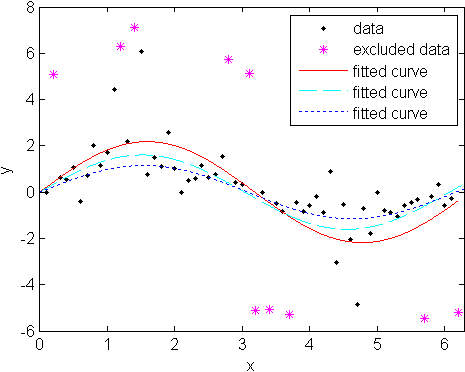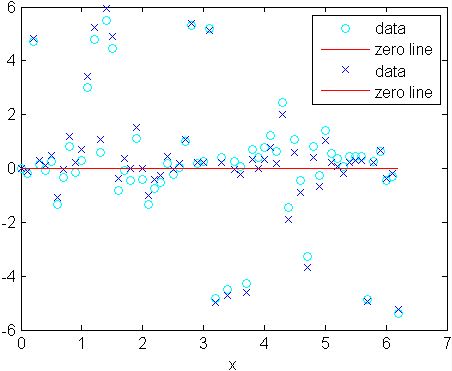### Example: Robust Fitting

1. Create a baseline sinusoidal signal:
```xdata = (0:0.1:2*pi)';
y0 = sin(xdata);```
2. Add noise to the signal with non-constant variance:
```% Response-dependent Gaussian noise
gnoise = y0.*randn(size(y0));

% Salt-and-pepper noise
spnoise = zeros(size(y0));
p = randperm(length(y0));
sppoints = p(1:round(length(p)/5));
spnoise(sppoints) = 5*sign(y0(sppoints));

ydata = y0 + gnoise + spnoise;```
3. Fit the noisy data with a baseline sinusoidal model:
```f = fittype('a*sin(b*x)');
fit1 = fit(xdata,ydata,f,'StartPoint',[1 1]);```
4. Identify “outliers” as points at a distance greater than 1.5 standard deviations from the baseline model, and refit the data with the outliers excluded:
```fdata = feval(fit1,xdata);
I = abs(fdata - ydata) > 1.5*std(ydata);
outliers = excludedata(xdata,ydata,'indices',I);

fit2 = fit(xdata,ydata,f,'StartPoint',[1 1],'Exclude',outliers);```
5. Compare the effect of excluding the outliers with the effect of giving them lower bisquare weight in a robust fit:
`fit3 = fit(xdata,ydata,f,'StartPoint',[1 1],'Robust','on');`
6. Plot the data, the outliers, and the results of the fits:
```plot(fit1,'r-',xdata,ydata,'k.',outliers,'m*')
hold on
plot(fit2,'c--')
plot(fit3,'b:')
xlim([0 2*pi])```7. Plot the residuals for the two fits considering outliers:
```figure
plot(fit2,xdata,ydata,'co','residuals')
hold on
plot(fit3,xdata,ydata,'bx','residuals')```

یادگیری :
یک سری دیتا داریم و می خئاهیم یک سری اطلاعات از آن استخراج کنیم
مثل رگرسیون که میتواند چند متغیره باشد

مقادیر ثابت نامعلوم که قصد برآور آنرا داریم

تابعی داریم که بر اساس آن برآورد را انجام میدهیم
تابع هدف ، امید ریاضی یک تابع دیگری است
q=
هدف پیدا کردن مقدار ماکزیمم یا مینی مم تابع g(c) هستامروز ۱۵ بهمن ۹۱

باشگاه دانشجویان ارشد مجازی امیرکبیر رو به آدرس http://vua.nadiran.com ایجاد کردم

تا محیطی باشه برای تبادل اطلاعات دوستان و مشارکت گروهی

موفق و سربلند باشید

محمد نادی

با کلیک روی آگهی زیر مبلغ 400 ریال به حساب من واریز می گردد

با کلیک روی آگهی زیر مبلغ 1000 ریال به حساب من واریز می گردد